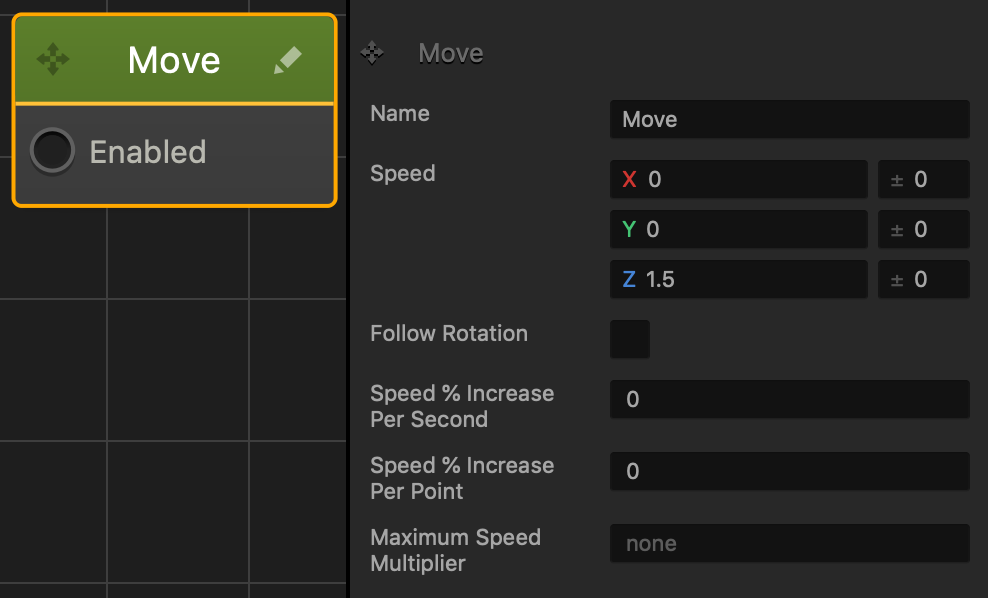## Move Node

This movement node allows the asset to travel in the direction specified in the axis values. See also Making Characters Jump and Objects Move, and Move Brainbox.• Speed
• Enter a numeric value in any of the axis fields to represent the movement direction and speed.
• The greater the number, the faster the asset will move.
• Direction
• To make an asset move:
• Forward, enter a positive value in the Z axis field.
• Backward, enter a negative value in the Z axis field.
• Right, enter a positive value in the axis field.
• left, enter a negative value in the axis field.
• Up, enter a positive value in the axis field.
• Down, enter a negative value in the Y axis field.
• Also depends on which direction the game camera is facing.
• If you put values in more than one axis, the asset will move in all specified directions.
• For example, if the Z and X axis fields contain values, the asset will move in a diagonal direction.
• Range
• To make the asset pick a random axis value, enter a value in any of the range fields to the right.
• The range value is both added and subtracted to create a minimum and maximum of possible outcomes.
• For example, if you enter a value of 100 in the Y axis field and 50 in its range value field, a value between 50 and 150 will be selected during gameplay.
|50———-100———-150|
If you don’t want the asset to rotate in a specific direction, delete the value in any of the axis fields.

The table below shows sample values in the X, Y, and Z axis with their speed and direction.

 X Y Z Sample Value 10 -50 20 Speed Slow Fast Medium Direction Right Down Forward

### Node Attributes

 Option Description Name Enter a custom name for this node, if needed. Speed Enter a numeric value in the axis fields to specify the speed and direction the asset will move in. The higher the number, the faster the asset will move. Enter a range value on the right to make the asset pick a random axis value. If there is no value in an axis field, then other nodes can affect this asset in those axes. This is useful when adding a Jump node to an asset. Follow Rotation To make the asset always face the direction it is moving, select this option. Speed % Increase per second Enter the percentage value to multiply the speed by a percentage after every second. For example, if you enter the following values: Speed—2 Speed % Increase Per Second—50 After every second, the speed will increase by 50% of its value or 1 in this example. Speed % Increase per point Enter the percentage of the speed value to multiply the speed for every point score in total. For example, if you enter the following values: Speed—3 Speed % Increase Per Point—50 Total Point score—5 For ever total point, the Speed will multiply by 50% or 1.5 in this example. With a Total Point score of 5, the speed will be increased 5 times to a total speed value of 10.5 Maximum Speed Multiplier Enter the maximum amount of times you want the speed to multiply by the Speed % attributes. If you don’t want a maximum speed multiplier, delete the value from the field until None is displayed. For example, if you enter the following values: Speed—3 Speed % Increase Per Point—50 Total Point score—100 Maximum Speed Multiplier—20 The speed will stop increasing after the score reaches a value of 20.

If needed, you can also customize this node. For details, see Customizing Nodes.